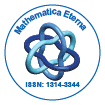# Mathematica EternaOpen Access

ISSN: 1314-3344

## Abstract

### Optimal Bounds for Neuman-S´andor Mean in Terms of the Convex Combination of Geometric and Quadratic Means

Liu Chunrong, Wang Jing

In this paper, we present the least value α and the greatest value β such that the double inequality αG(a, b) + (1 − α)Q(a, b) < M(a, b) < βG(a, b) + (1 − β)Q(a, b) holds for all a, b > 0 with a 6= b, where G(a,b), M(a,b) and Q(a,b) are respectively the geometric, Neuman-S´andor and quadratic means of a and b.

Top